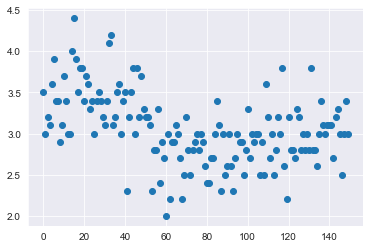# Write programs to illustrate the different plotting data distributions like, Univariate Distributions, Bivariate Distributions.

Univariate Data:

This type of data consists of only one variable. The analysis of Univariate data is thus the simplest form of analysis since the information deals with only one quantity that changes. It does not deal with causes or relationships and the main purpose of the analysis is to describe the data and find patterns that exist within it. The example of a Univariate data can be height. For this example, Download CSV file here

Example program

import pandas as pd
import numpy as np
import matplotlib.pyplot as plt
%matplotlib inline
import seaborn as sns #SEABORN IMPORTING
plt.scatter(df.index,df['sepal.width'])
plt.show()

Output:-Bivariate data

This type of data involves two different variables. The analysis of this type of data deals with causes and relationships and the analysis is done to find out the relationship among the two variables. Example of Bivariate data can be temperature and ice cream sales in summer season.

Suppose the temperature and ice cream sales are the two variables of a bivariate data. Here, the relationship is visible from the table that temperature and sales are directly proportional to each other and thus related because as the temperature increases, the sales also increase. Thus bivariate data analysis involves comparisons, relationships, causes and explanations. These variables are often plotted on X and Y axis on the graph for better understanding of data and one of these variables is independent while the other is dependent.

More

More

MFCS
COA
PL-CG
DBMS
OPERATING SYSTEM
SOFTWARE ENG
DSA
TOC-CD
ARTIFICIAL INT

More

More

More

More
Top